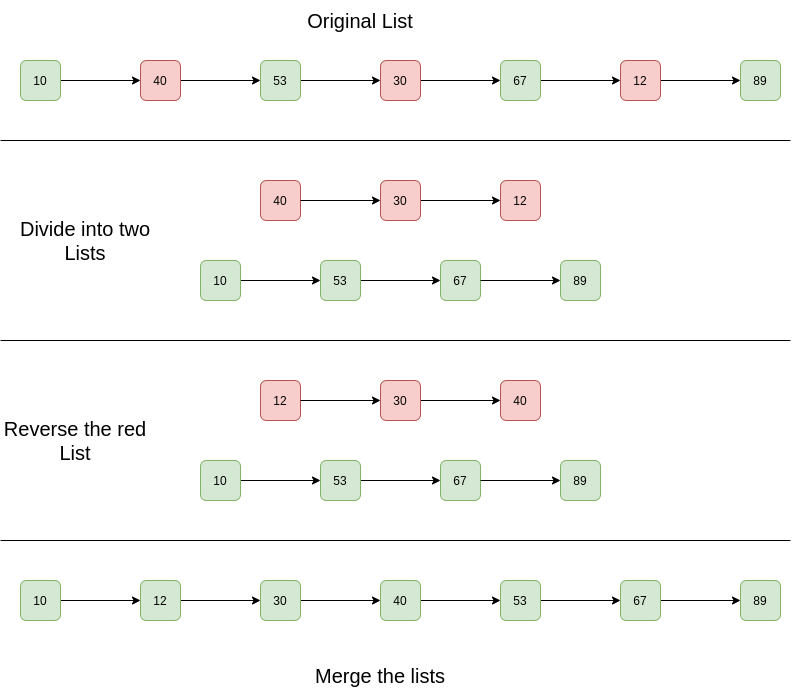GeeksforGeeks App
Open AppBrowser
Continue

# Java Program For Sorting A Linked List That Is Sorted Alternating Ascending And Descending Orders

Given a Linked List. The Linked List is in alternating ascending and descending orders. Sort the list efficiently.

Example:

```Input List: 10 -> 40 -> 53 -> 30 -> 67 -> 12 -> 89 -> NULL
Output List: 10 -> 12 -> 30 -> 40 -> 53 -> 67 -> 89 -> NULL

Input List: 1 -> 4 -> 3 -> 2 -> 5 -> NULL
Output List: 1 -> 2 -> 3 -> 4 -> 5 -> NULL```

Simple Solution:
Approach: The basic idea is to apply to merge sort on the linked list.
Complexity Analysis:

• Time Complexity: The merge sort of linked list takes O(n log n) time. In the merge sort tree, the height is log n. Sorting each level will take O(n) time. So time complexity is O(n log n).
• Auxiliary Space: O(n log n), In the merge sort tree the height is log n. Storing each level will take O(n) space. So space complexity is O(n log n).

Efficient Solution:
Approach:

1. Separate two lists.
2. Reverse the one in descending order
3. Merge both lists.

Diagram:Below are the implementations of the above algorithm:

## Java

 `// Java program to sort a linked list``// that is alternatively sorted in``// increasing and decreasing order``import` `java.io.*;` `public` `class` `LinkedList {``    ``// head of list``    ``Node head;` `    ``// Linked list Node``    ``class` `Node {``        ``int` `data;``        ``Node next;``        ``Node(``int` `d)``        ``{``            ``data = d;``            ``next = ``null``;``        ``}``    ``}` `    ``Node newNode(``int` `key) { ``return` `new` `Node(key); }` `    ``/* This is the main function that sorts``       ``the linked list.*/``    ``void` `sort()``    ``{``        ``/* Create 2 dummy nodes and initialise``           ``as heads of linked lists */``        ``Node Ahead = ``new` `Node(``0``), Dhead = ``new` `Node(``0``);` `        ``// Split the list into lists``        ``splitList(Ahead, Dhead);` `        ``Ahead = Ahead.next;``        ``Dhead = Dhead.next;` `        ``// Reverse the descending list``        ``Dhead = reverseList(Dhead);` `        ``// Merge the 2 linked lists``        ``head = mergeList(Ahead, Dhead);``    ``}` `    ``// Function to reverse the linked list``    ``Node reverseList(Node Dhead)``    ``{``        ``Node current = Dhead;``        ``Node prev = ``null``;``        ``Node next;``        ``while` `(current != ``null``) {``            ``next = current.next;``            ``current.next = prev;``            ``prev = current;``            ``current = next;``        ``}``        ``Dhead = prev;``        ``return` `Dhead;``    ``}` `    ``// Function to print linked list``    ``void` `printList()``    ``{``        ``Node temp = head;``        ``while` `(temp != ``null``) {``            ``System.out.print(temp.data + ``" "``);``            ``temp = temp.next;``        ``}``        ``System.out.println();``    ``}` `    ``// A utility function to merge``    ``// two sorted linked lists``    ``Node mergeList(Node head1, Node head2)``    ``{``        ``// Base cases``        ``if` `(head1 == ``null``)``            ``return` `head2;``        ``if` `(head2 == ``null``)``            ``return` `head1;` `        ``Node temp = ``null``;``        ``if` `(head1.data < head2.data) {``            ``temp = head1;``            ``head1.next = mergeList(head1.next, head2);``        ``}``        ``else` `{``            ``temp = head2;``            ``head2.next = mergeList(head1, head2.next);``        ``}``        ``return` `temp;``    ``}` `    ``// This function alternatively splits``    ``// a linked list with head as head into two:``    ``// For example, 10->20->30->15->40->7 is``    ``// splitted into 10->30->40 and 20->15->7``    ``// "Ahead" is reference to head of ascending``    ``// linked list``    ``// "Dhead" is reference to head of descending``    ``// linked list``    ``void` `splitList(Node Ahead, Node Dhead)``    ``{``        ``Node ascn = Ahead;``        ``Node dscn = Dhead;``        ``Node curr = head;` `        ``// Link alternate nodes` `        ``while` `(curr != ``null``) {``            ``// Link alternate nodes in``            ``// ascending order``            ``ascn.next = curr;``            ``ascn = ascn.next;``            ``curr = curr.next;` `            ``if` `(curr != ``null``) {``                ``dscn.next = curr;``                ``dscn = dscn.next;``                ``curr = curr.next;``            ``}``        ``}` `        ``ascn.next = ``null``;``        ``dscn.next = ``null``;``    ``}` `    ``// Driver code``    ``public` `static` `void` `main(String args[])``    ``{``        ``LinkedList llist = ``new` `LinkedList();``        ``llist.head = llist.newNode(``10``);``        ``llist.head.next = llist.newNode(``40``);``        ``llist.head.next.next = llist.newNode(``53``);``        ``llist.head.next.next.next = llist.newNode(``30``);``        ``llist.head.next.next.next.next = llist.newNode(``67``);``        ``llist.head.next.next.next.next.next``            ``= llist.newNode(``12``);``        ``llist.head.next.next.next.next.next.next``            ``= llist.newNode(``89``);` `        ``System.out.println(``"Given linked list"``);``        ``llist.printList();` `        ``llist.sort();` `        ``System.out.println(``"Sorted linked list"``);``        ``llist.printList();``    ``}``}``// This code is contributed by Rajat Mishra`

Output

```Given linked list
10 40 53 30 67 12 89
10 12 30 40 53 67 89
```

Complexity Analysis:

• Time Complexity: O(n).
One traversal is needed to separate the list and reverse them. The merging of sorted lists takes O(n) time.
• Auxiliary Space: O(1).
No extra space is required.

Please refer complete article on Sort a linked list that is sorted alternating ascending and descending orders? for more details!

My Personal Notes arrow_drop_up